## Python Tutorial

Python HOME Python Intro Python Get Started Python Syntax Python Comments Python Variables Python Data Types Python Numbers Python Casting Python Strings Python Booleans Python Operators Python Lists Python Tuples Python Sets Python Dictionaries Python If...Else Python While Loops Python For Loops Python Functions Python Lambda Python Arrays Python Classes/Objects Python Inheritance Python Iterators Python Polymorphism Python Scope Python Modules Python Dates Python Math Python JSON Python RegEx Python PIP Python Try...Except Python User Input Python String Formatting

## File Handling

Python File Handling Python Read Files Python Write/Create Files Python Delete Files

## Python Modules

NumPy Tutorial Pandas Tutorial SciPy Tutorial Django Tutorial

## Python Matplotlib

Matplotlib Intro Matplotlib Get Started Matplotlib Pyplot Matplotlib Plotting Matplotlib Markers Matplotlib Line Matplotlib Labels Matplotlib Grid Matplotlib Subplot Matplotlib Scatter Matplotlib Bars Matplotlib Histograms Matplotlib Pie Charts

## Machine Learning

Getting Started Mean Median Mode Standard Deviation Percentile Data Distribution Normal Data Distribution Scatter Plot Linear Regression Polynomial Regression Multiple Regression Scale Train/Test Decision Tree Confusion Matrix Hierarchical Clustering Logistic Regression Grid Search Categorical Data K-means Bootstrap Aggregation Cross Validation AUC - ROC Curve K-nearest neighbors

## Python MySQL

MySQL Get Started MySQL Create Database MySQL Create Table MySQL Insert MySQL Select MySQL Where MySQL Order By MySQL Delete MySQL Drop Table MySQL Update MySQL Limit MySQL Join

## Python MongoDB

MongoDB Get Started MongoDB Create Database MongoDB Create Collection MongoDB Insert MongoDB Find MongoDB Query MongoDB Sort MongoDB Delete MongoDB Drop Collection MongoDB Update MongoDB Limit

## Python Reference

Python Overview Python Built-in Functions Python String Methods Python List Methods Python Dictionary Methods Python Tuple Methods Python Set Methods Python File Methods Python Keywords Python Exceptions Python Glossary

## Module Reference

Random Module Requests Module Statistics Module Math Module cMath Module

## Python How To

Remove List Duplicates Reverse a String Add Two Numbers

## Python Examples

Python Examples Python Compiler Python Exercises Python Quiz Python Bootcamp Python Certificate

# Matplotlib Scatter

## Creating Scatter Plots

With Pyplot, you can use the `scatter()` function to draw a scatter plot.

The `scatter()` function plots one dot for each observation. It needs two arrays of the same length, one for the values of the x-axis, and one for values on the y-axis:

### Example

A simple scatter plot:

import matplotlib.pyplot as plt
import numpy as np

x = np.array([5,7,8,7,2,17,2,9,4,11,12,9,6])
y = np.array([99,86,87,88,111,86,103,87,94,78,77,85,86])

plt.scatter(x, y)
plt.show()

### Result: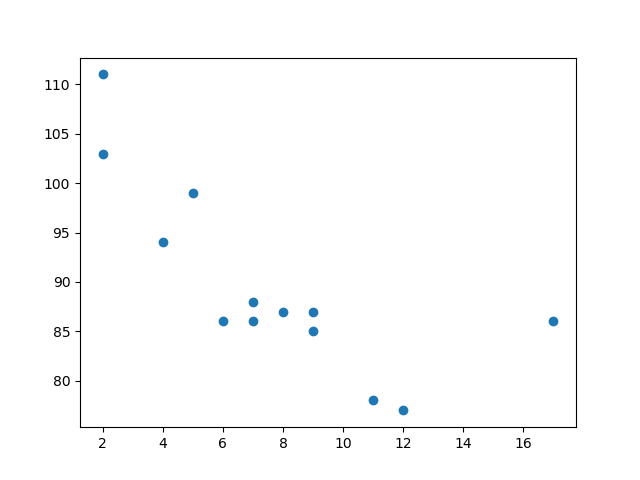Try it Yourself »

The observation in the example above is the result of 13 cars passing by.

The X-axis shows how old the car is.

The Y-axis shows the speed of the car when it passes.

Are there any relationships between the observations?

It seems that the newer the car, the faster it drives, but that could be a coincidence, after all we only registered 13 cars.

## Compare Plots

In the example above, there seems to be a relationship between speed and age, but what if we plot the observations from another day as well? Will the scatter plot tell us something else?

### Example

Draw two plots on the same figure:

import matplotlib.pyplot as plt
import numpy as np

#day one, the age and speed of 13 cars:
x = np.array([5,7,8,7,2,17,2,9,4,11,12,9,6])
y = np.array([99,86,87,88,111,86,103,87,94,78,77,85,86])
plt.scatter(x, y)

#day two, the age and speed of 15 cars:
x = np.array([2,2,8,1,15,8,12,9,7,3,11,4,7,14,12])
y = np.array([100,105,84,105,90,99,90,95,94,100,79,112,91,80,85])
plt.scatter(x, y)

plt.show()

### Result: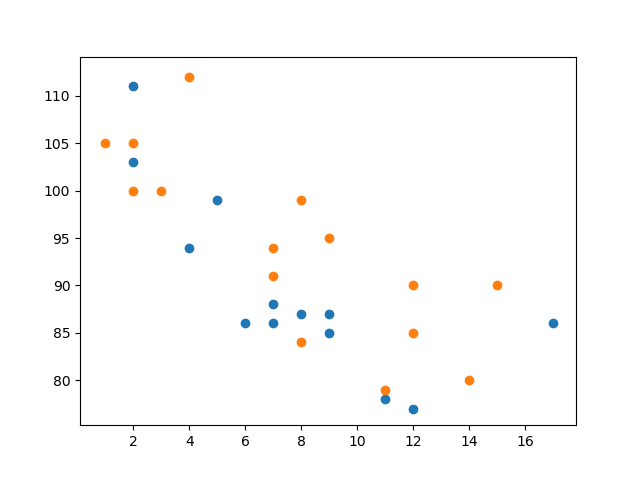Try it Yourself »

Note: The two plots are plotted with two different colors, by default blue and orange, you will learn how to change colors later in this chapter.

By comparing the two plots, I think it is safe to say that they both gives us the same conclusion: the newer the car, the faster it drives.

## Colors

You can set your own color for each scatter plot with the `color` or the `c` argument:

### Example

Set your own color of the markers:

import matplotlib.pyplot as plt
import numpy as np

x = np.array([5,7,8,7,2,17,2,9,4,11,12,9,6])
y = np.array([99,86,87,88,111,86,103,87,94,78,77,85,86])
plt.scatter(x, y, color = 'hotpink')

x = np.array([2,2,8,1,15,8,12,9,7,3,11,4,7,14,12])
y = np.array([100,105,84,105,90,99,90,95,94,100,79,112,91,80,85])
plt.scatter(x, y, color = '#88c999')

plt.show()

### Result: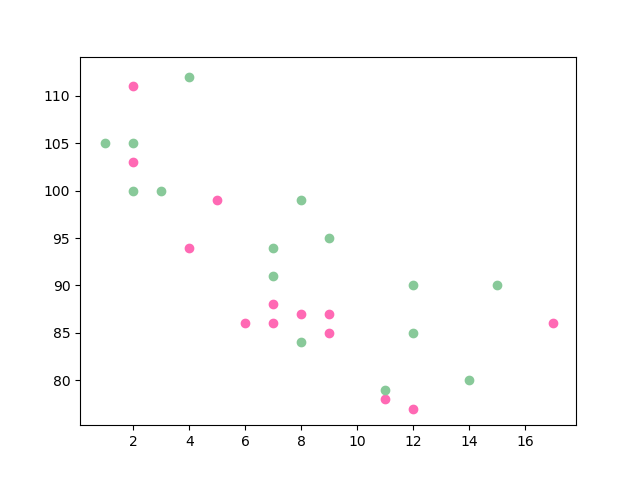Try it Yourself »

## Color Each Dot

You can even set a specific color for each dot by using an array of colors as value for the `c` argument:

Note: You cannot use the `color` argument for this, only the `c` argument.

### Example

Set your own color of the markers:

import matplotlib.pyplot as plt
import numpy as np

x = np.array([5,7,8,7,2,17,2,9,4,11,12,9,6])
y = np.array([99,86,87,88,111,86,103,87,94,78,77,85,86])
colors = np.array(["red","green","blue","yellow","pink","black","orange","purple","beige","brown","gray","cyan","magenta"])

plt.scatter(x, y, c=colors)

plt.show()

### Result: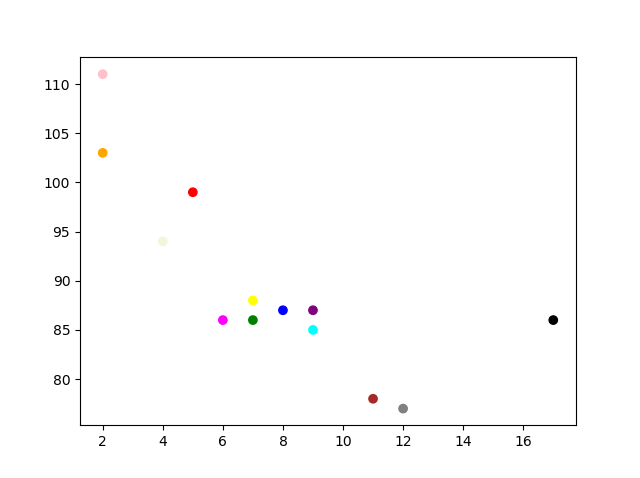Try it Yourself »

## ColorMap

The Matplotlib module has a number of available colormaps.

A colormap is like a list of colors, where each color has a value that ranges from 0 to 100.

Here is an example of a colormap:This colormap is called 'viridis' and as you can see it ranges from 0, which is a purple color, up to 100, which is a yellow color.

### How to Use the ColorMap

You can specify the colormap with the keyword argument `cmap` with the value of the colormap, in this case `'viridis'` which is one of the built-in colormaps available in Matplotlib.

In addition you have to create an array with values (from 0 to 100), one value for each point in the scatter plot:

### Example

Create a color array, and specify a colormap in the scatter plot:

import matplotlib.pyplot as plt
import numpy as np

x = np.array([5,7,8,7,2,17,2,9,4,11,12,9,6])
y = np.array([99,86,87,88,111,86,103,87,94,78,77,85,86])
colors = np.array([0, 10, 20, 30, 40, 45, 50, 55, 60, 70, 80, 90, 100])

plt.scatter(x, y, c=colors, cmap='viridis')

plt.show()

### Result: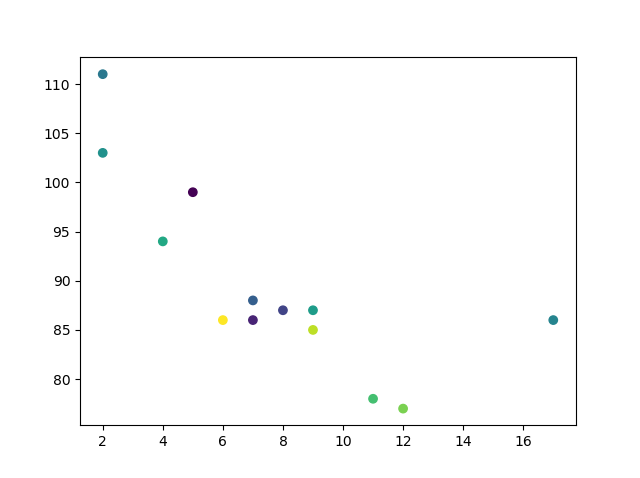Try it Yourself »

You can include the colormap in the drawing by including the `plt.colorbar()` statement:

### Example

Include the actual colormap:

import matplotlib.pyplot as plt
import numpy as np

x = np.array([5,7,8,7,2,17,2,9,4,11,12,9,6])
y = np.array([99,86,87,88,111,86,103,87,94,78,77,85,86])
colors = np.array([0, 10, 20, 30, 40, 45, 50, 55, 60, 70, 80, 90, 100])

plt.scatter(x, y, c=colors, cmap='viridis')

plt.colorbar()

plt.show()

### Result: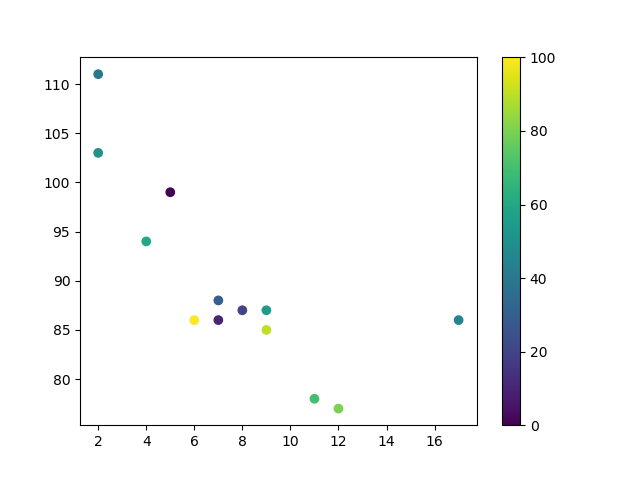Try it Yourself »

### Available ColorMaps

You can choose any of the built-in colormaps:

Name   Reverse
Accent Try it »   Accent_r Try it »
Blues Try it »   Blues_r Try it »
BrBG Try it »   BrBG_r Try it »
BuGn Try it »   BuGn_r Try it »
BuPu Try it »   BuPu_r Try it »
CMRmap Try it »   CMRmap_r Try it »
Dark2 Try it »   Dark2_r Try it »
GnBu Try it »   GnBu_r Try it »
Greens Try it »   Greens_r Try it »
Greys Try it »   Greys_r Try it »
OrRd Try it »   OrRd_r Try it »
Oranges Try it »   Oranges_r Try it »
PRGn Try it »   PRGn_r Try it »
Paired Try it »   Paired_r Try it »
Pastel1 Try it »   Pastel1_r Try it »
Pastel2 Try it »   Pastel2_r Try it »
PiYG Try it »   PiYG_r Try it »
PuBu Try it »   PuBu_r Try it »
PuBuGn Try it »   PuBuGn_r Try it »
PuOr Try it »   PuOr_r Try it »
PuRd Try it »   PuRd_r Try it »
Purples Try it »   Purples_r Try it »
RdBu Try it »   RdBu_r Try it »
RdGy Try it »   RdGy_r Try it »
RdPu Try it »   RdPu_r Try it »
RdYlBu Try it »   RdYlBu_r Try it »
RdYlGn Try it »   RdYlGn_r Try it »
Reds Try it »   Reds_r Try it »
Set1 Try it »   Set1_r Try it »
Set2 Try it »   Set2_r Try it »
Set3 Try it »   Set3_r Try it »
Spectral Try it »   Spectral_r Try it »
Wistia Try it »   Wistia_r Try it »
YlGn Try it »   YlGn_r Try it »
YlGnBu Try it »   YlGnBu_r Try it »
YlOrBr Try it »   YlOrBr_r Try it »
YlOrRd Try it »   YlOrRd_r Try it »
afmhot Try it »   afmhot_r Try it »
autumn Try it »   autumn_r Try it »
binary Try it »   binary_r Try it »
bone Try it »   bone_r Try it »
brg Try it »   brg_r Try it »
bwr Try it »   bwr_r Try it »
cividis Try it »   cividis_r Try it »
cool Try it »   cool_r Try it »
coolwarm Try it »   coolwarm_r Try it »
copper Try it »   copper_r Try it »
cubehelix Try it »   cubehelix_r Try it »
flag Try it »   flag_r Try it »
gist_earth Try it »   gist_earth_r Try it »
gist_gray Try it »   gist_gray_r Try it »
gist_heat Try it »   gist_heat_r Try it »
gist_ncar Try it »   gist_ncar_r Try it »
gist_rainbow Try it »   gist_rainbow_r Try it »
gist_stern Try it »   gist_stern_r Try it »
gist_yarg Try it »   gist_yarg_r Try it »
gnuplot Try it »   gnuplot_r Try it »
gnuplot2 Try it »   gnuplot2_r Try it »
gray Try it »   gray_r Try it »
hot Try it »   hot_r Try it »
hsv Try it »   hsv_r Try it »
inferno Try it »   inferno_r Try it »
jet Try it »   jet_r Try it »
magma Try it »   magma_r Try it »
nipy_spectral Try it »   nipy_spectral_r Try it »
ocean Try it »   ocean_r Try it »
pink Try it »   pink_r Try it »
plasma Try it »   plasma_r Try it »
prism Try it »   prism_r Try it »
rainbow Try it »   rainbow_r Try it »
seismic Try it »   seismic_r Try it »
spring Try it »   spring_r Try it »
summer Try it »   summer_r Try it »
tab10 Try it »   tab10_r Try it »
tab20 Try it »   tab20_r Try it »
tab20b Try it »   tab20b_r Try it »
tab20c Try it »   tab20c_r Try it »
terrain Try it »   terrain_r Try it »
twilight Try it »   twilight_r Try it »
twilight_shifted Try it »   twilight_shifted_r Try it »
viridis Try it »   viridis_r Try it »
winter Try it »   winter_r Try it »

## Size

You can change the size of the dots with the `s` argument.

Just like colors, make sure the array for sizes has the same length as the arrays for the x- and y-axis:

### Example

Set your own size for the markers:

import matplotlib.pyplot as plt
import numpy as np

x = np.array([5,7,8,7,2,17,2,9,4,11,12,9,6])
y = np.array([99,86,87,88,111,86,103,87,94,78,77,85,86])
sizes = np.array([20,50,100,200,500,1000,60,90,10,300,600,800,75])

plt.scatter(x, y, s=sizes)

plt.show()

### Result: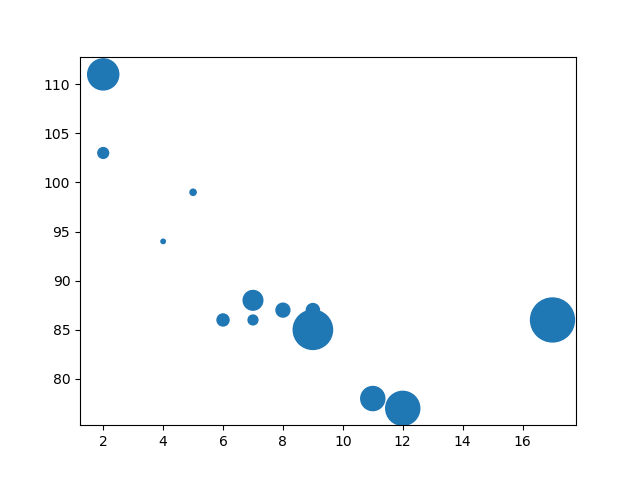Try it Yourself »

## Alpha

You can adjust the transparency of the dots with the `alpha` argument.

Just like colors, make sure the array for sizes has the same length as the arrays for the x- and y-axis:

### Example

Set your own size for the markers:

import matplotlib.pyplot as plt
import numpy as np

x = np.array([5,7,8,7,2,17,2,9,4,11,12,9,6])
y = np.array([99,86,87,88,111,86,103,87,94,78,77,85,86])
sizes = np.array([20,50,100,200,500,1000,60,90,10,300,600,800,75])

plt.scatter(x, y, s=sizes, alpha=0.5)

plt.show()

### Result: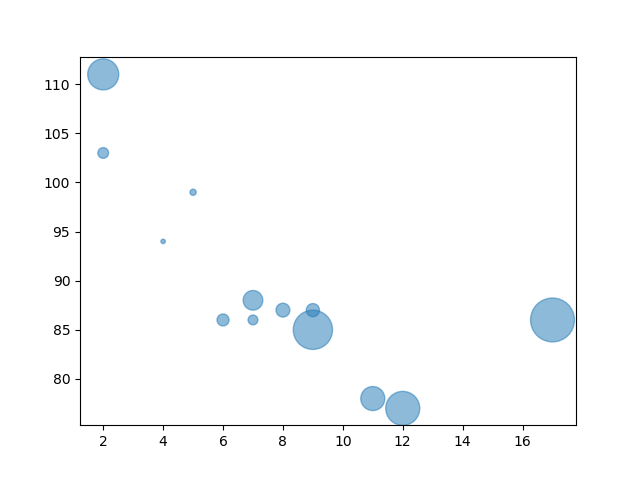Try it Yourself »

## Combine Color Size and Alpha

You can combine a colormap with different sizes of the dots. This is best visualized if the dots are transparent:

### Example

Create random arrays with 100 values for x-points, y-points, colors and sizes:

import matplotlib.pyplot as plt
import numpy as np

x = np.random.randint(100, size=(100))
y = np.random.randint(100, size=(100))
colors = np.random.randint(100, size=(100))
sizes = 10 * np.random.randint(100, size=(100))

plt.scatter(x, y, c=colors, s=sizes, alpha=0.5, cmap='nipy_spectral')

plt.colorbar()

plt.show()

### Result: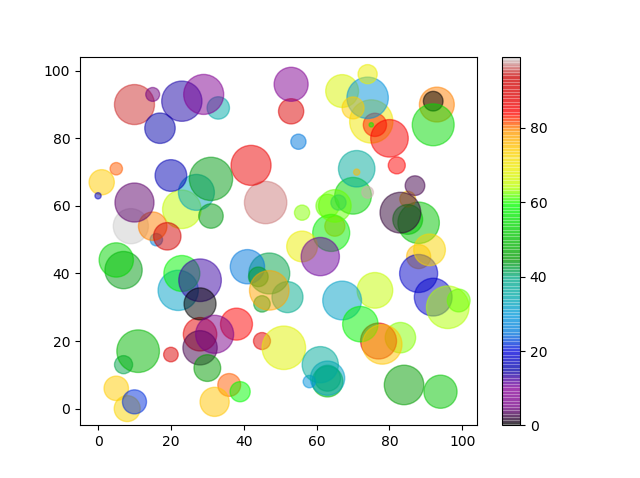Try it Yourself »

W3Schools is optimized for learning and training. Examples might be simplified to improve reading and learning. Tutorials, references, and examples are constantly reviewed to avoid errors, but we cannot warrant full correctness of all content. While using W3Schools, you agree to have read and accepted our terms of use, cookie and privacy policy.